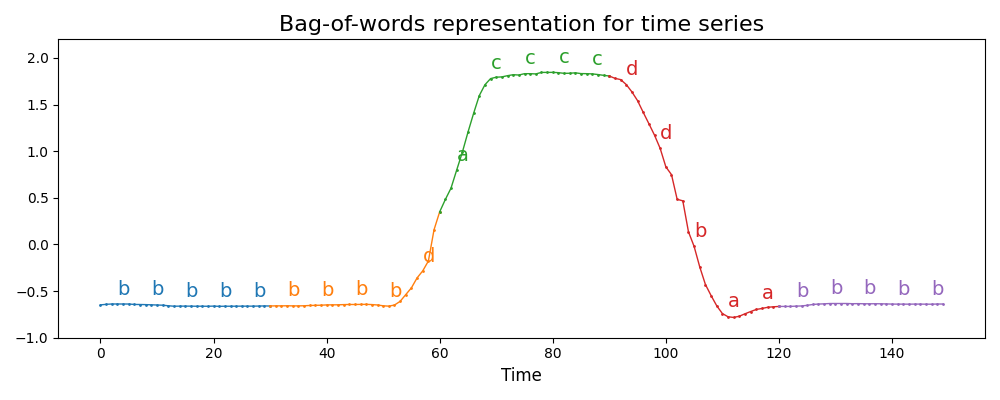# Bag of Words¶

Time series are often transformed into sequences of symbols. Bag-of-words approaches are then used to extract features from these sequences. Identical back-to-back words can be discarded using the `numerosity_reduction` parameter.

This example illustrates the transformation in a particular setting: the step of the sliding window is equal to the size of the sliding window, making the subseries non-overlapping. It is common to use a step of 1 for the sliding window, which is the default behavior. It is implemented as `pyts.bag_of_words.BagOfWords`.```# Author: Johann Faouzi <johann.faouzi@gmail.com>

import matplotlib.pyplot as plt
import numpy as np
from pyts.bag_of_words import BagOfWords

# Load the dataset and perform the transformation
X, _, _, _ = load_gunpoint(return_X_y=True)
window_size, word_size = 30, 5
bow = BagOfWords(window_size=window_size, word_size=word_size,
window_step=window_size, numerosity_reduction=False)
X_bow = bow.transform(X)

# Plot the considered subseries
plt.figure(figsize=(10, 4))
splits_series = np.linspace(0, X.shape, 1 + X.shape // window_size,
dtype='int64')
for start, end in zip(splits_series[:-1],
np.clip(splits_series[1:] + 1, 0, X.shape)):
plt.plot(np.arange(start, end), X[0, start:end], 'o-', lw=1, ms=1)

# Plot the corresponding letters
splits_letters = np.linspace(0, X.shape,
1 + word_size * X.shape // window_size)
splits_letters = (
(splits_letters[:-1] + splits_letters[1:]) / 2).astype('int64')
for i, (x, text) in enumerate(zip(splits_letters, X_bow.replace(' ', ''))):
plt.text(x, X[0, x] + 0.1, text, color="C{}".format(i // 5), fontsize=14)

plt.ylim((-1, 2.2))
plt.xlabel('Time', fontsize=12)
plt.title('Bag-of-words representation for time series', fontsize=16)
plt.tight_layout()
plt.show()
```

Total running time of the script: ( 0 minutes 0.493 seconds)

Gallery generated by Sphinx-Gallery